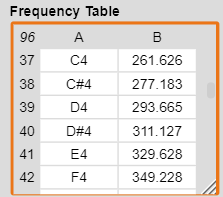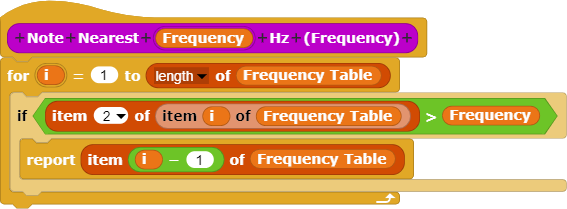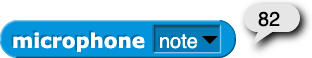# Matching Note with Frequency?

I created a table of musical notes and corresponding frequencies:I would like to create a block that reports the musical note that is nearest a specified frequency. One approach would to create a loop and check each frequency in the table until the closest one is identified. Since there are only eight octaves of notes in the table (i.e., 96 items), that might not be an unreasonable approach. However, is there a more elegant strategy?ok

you could try incorporating some sort of binary search

or you could estimate the index of the frequency in the table. since musical scales are exponential, you’ll probably have to use logarithms to do this.

I would think it should be possible to come up with a Freq -> Note equation

I think @warped_wart_wars is the local maths enthusiast so maybe they'd come up with oneyeah it’d be the inverse of the function here right?

Oh they have the inverse function there already.

Yep - that looks like enough info to get started

Use equation to find note number and then look that up in a simple list of notes

Thanks!

If you load the audio comp library, there's a note from hz block, which might be what you're looking for. I created a block that can translate a note number to a note name, so here it is.

I included a few more blocks in the project that might be useful.

not really important but whether you say, for example, C# or Db is dependent upon the scale. So the notes in the B major scale would be (getting this from memory i may be incorrect) B C# D# E F# G# A#, and not like B Db D# E F# G# A#, because in the latter D appears twice. In your situation, however, there isn’t really a defined scale so you can just do whatever you want I guess. Which is why I said this isn’t really important.

This is very nice. Thanks for sharing this.

You're welcome.

1. Yeah.
2. I don't know much about the mathematics of music.

I always use the built-in note reporter:and one of my favorite little quick projects is to connect it with the play frequency command inside a forever loop to make an auto-tune "Cher" effect for whistling into my headset microphone.

But you need the Math behind that, because you're not getting the frequency from the microphone right?
I'm using this formula for it:

        this.note = Math.round(
12 * (Math.log(this.pitch / 440) / Math.log(2))
) + 69;


Does this help?

 oh right! I forgot all about the reporter in the audio comp library, thanks, @ego-lay_atman-bay !

In Snap!, that'd be

right?

(project)

(lg there is $$\log_2$$, and $$\log_ab={\log_nb\over\log_na}$$.)

This is great stuff ... the microphone block is terrific.

$$\log_2$$ is totally what you want, since the ratio of frequencies of adjacent piano keys is $$^{12}\sqrt 2$$. So the log of the ratio is 1/12.

There's probably a better way of doing that, but I can't think of one.

Of formatting the 12th root of 2? $$\sqrt2$$ gives $$\sqrt2$$. I was just too lazy to look it up. I'm embarrassed to say that I've forgotten how to make the 12 smaller than the 2 in LaTeX.

Yeah.

$$\sqrt2$$ ... it works!